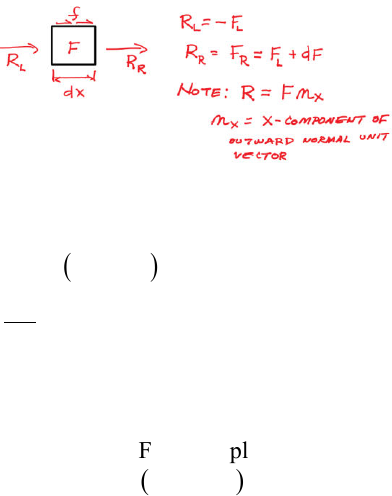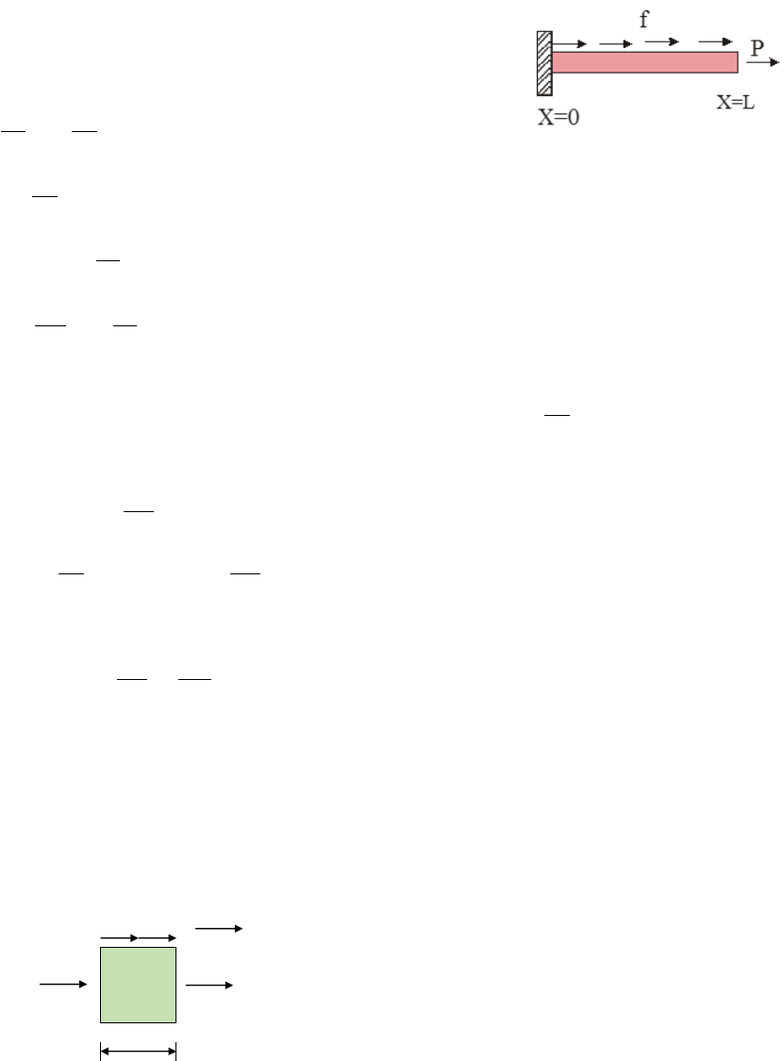Study Guides (390,000)
US (220,000)
TAMU (3,000)
AERO (10)
Final

# AERO 306 Lecture 15: 1_uniaxialRodExam

Department
Aerospace Engineering
Course Code
AERO 306
Professor
John Whitcomb
Study Guide
Final

This preview shows pages 1-3. to view the full 21 pages of the document.
C:\Users\cloudconvert\server\files\119\172\7\387d5855-1e5b-433a-8159-
Chapter 2
Approximate Analysis of Uniaxial
Bars and Beams

Only pages 1-3 are available for preview. Some parts have been intentionally blurred.C:\Users\cloudconvert\server\files\119\172\7\387d5855-1e5b-433a-8159-
The goal now is to develop a systematic procedure for obtaining approximate solutions. Uniaxial
bars and beams will be used to illustrate the basic concepts. We will begin with the uniaxial bar.
Analysis of a Uniaxial Rod
Solution of differential equations
To refresh your memory, we will begin by solving a uniaxial bar problem using just differential
equations.
The equilibrium equation can be derived by imposing equilibrium on a differential slice of the
bar as follows.
Summing forces gives
( )
0
0
LL
F F dF fdx
dF f
dx
− + + + =
+=
This equation is valid for any uniaxial rod even if the cross sectional area or material properties
are functions of x. Constitutive relations enter the picture when we express F in terms of
displacement. For example, if the material is thermoelastic, then we would substitute
( )
F A AE T
 
= = − 
Warning:
F = internal force, not applied force (Remember Cauchy’s formula)

Only pages 1-3 are available for preview. Some parts have been intentionally blurred.C:\Users\cloudconvert\server\files\119\172\7\387d5855-1e5b-433a-8159-
Now let’s solve a simple problem.
1
2
12
2
12
Solve differential equation by integrating two times
Assume: EA constant
f=constant
no thermal effects
2
1
2
d du
EA f
dx dx
du
EA fx C
dx
x
EAu f C x C
x
u f C x C
EA
=

=−


= − +
= − + +

= + +


Now impose the boundary conditions
(0) 0
xL
du
u and EA P
dx =

==


to determine the
integration constants.
( )
( )
22
1
1
2
1
1) (0) 0 (0 0 ) 0 0
1
2)
1 This is the exact solution.
2
xL
u C C
EA
du
EA P EA fL C P
dx EA
C P fL
fx
u P fL x
EA
=
= = + + = = =

= = − + =


= = +

 = + +


Further thought
Assume some variation of temperature along the length and re-solve this problem.
Derivation of Virtual Work
There are various ways to derive the virtual work equation for a uniaxial bar. This document
gives one version here and two more in the appendix to this file. First consider a differential
element of the bar in the equilibrium state.
-F
F + dF
dx
f
u